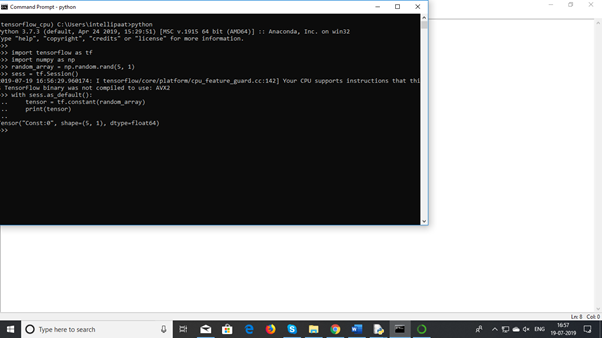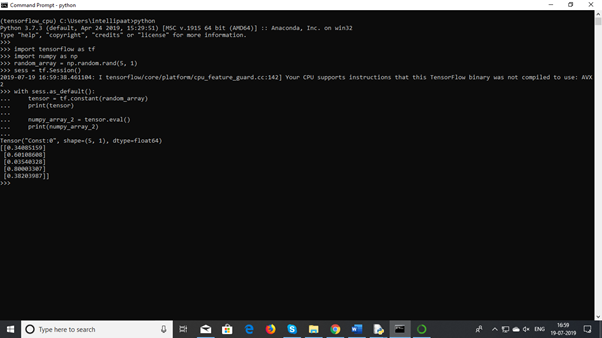# How to convert numpy arrays to standard TensorFlow format?

1 view

I have two numpy arrays:

• One that contains captcha images
• Another that contains the corresponding labels (in one-hot vector format)

I want to load these into TensorFlow so I can classify them using a neural network. How can this be done?

What shape do the numpy arrays need to have?

Additional Info - My images are 60 (height) by 160 (width) pixels each and each of them have 5 alphanumeric characters. Here is a sample image:Each label is a 5 by 62 array.

by (8k points)
edited by

Create a file convert.py with the following code:

import tensorflow as tf

import numpy as np

random_array = np.random.rand(5, 1)

sess = tf.Session()

with sess.as_default():

tensor = tf.constant(random_array)

print(tensor)By using tf.Session() with an input array of random numbers numpy array can be converted into tensors with tf.constant. Conversely, Tensors can be converted into numpy array with tensor.eval().

numpy_array_2 = tensor.eval()